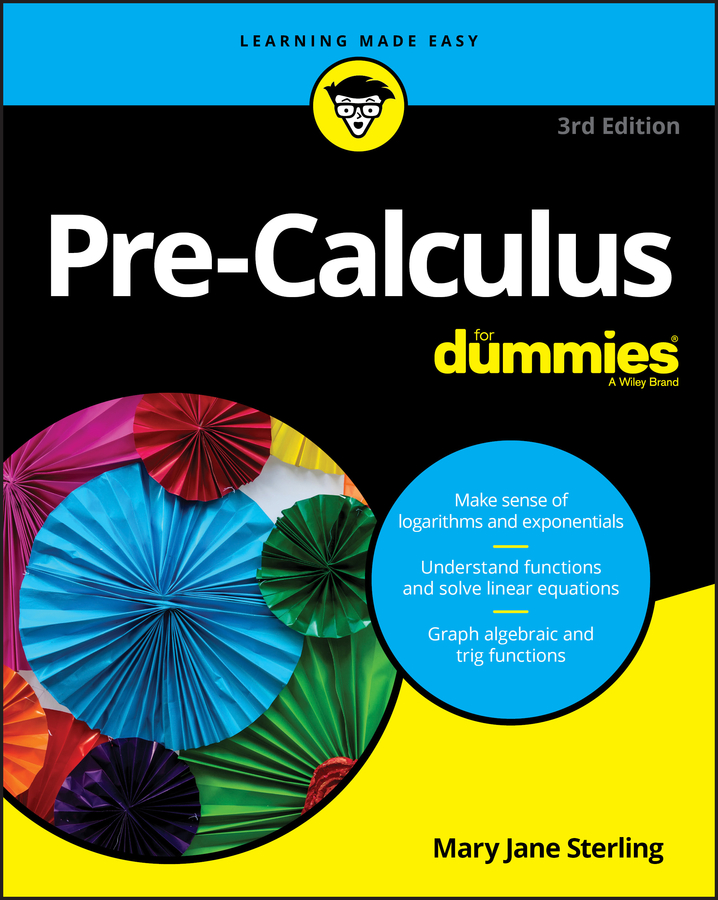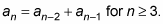##### Pre-Calculus For DummiesA recursive sequence is an arithmetic sequence in which each term depends on the term(s) before it; the Fibonacci sequence is a well-known example. When your pre-calculus teacher asks you to find any term in a recursive sequence, you use the given term (at least one term, usually the first, is given) and the given formula that allows you to find the other terms in the sequence.

You can recognize recursive sequences because the given formula typically has an (the nth term of the sequence) as well as an – 1 (the term before the nth term of the sequence). In these sequences, you're given a formula (a different one for each sequence), and the directions ask you to find the terms of the sequence.

For example, the most famous recursive sequence is the Fibonacci sequence, in which each term after the second term is defined as the sum of the two terms before it. The first term of this sequence is 1, and the second term is 1 also. The formula for the Fibonacci sequence isSo if you were asked to find the next three terms of the sequence, you'd have to use the formula as follows:

a3 = a3 – 2 + a3 – 1 = a1 + a2 = 1 + 1 = 2

a4 = a4 – 2 + a4 – 1 = a2 + a3 = 1 + 2 = 3

a5 = a5 – 2 + a5 – 1 = a3 + a4 = 2 + 3 = 5

The first ten terms of this sequence are 1, 1, 2, 3, 5, 8, 13, 21, 34, 55. It is very famous because many things in the natural world follow the pattern of the Fibonacci sequence. For examples, the florets in the head of a sunflower form two oppositely directed spirals, 55 of them clockwise and 34 counterclockwise; lilies and irises both have 3 petals; buttercups have 5 petals; and corn marigolds have 13 petals. Seeds of coneflowers and sunflowers have also been observed to follow the same pattern as the Fibonacci sequence. Pine cones and cauliflower also follow this pattern.